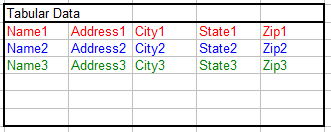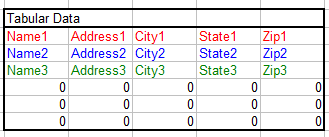Column To Table

This page describes formulas you can use to transform a column of data into a table of rows and columns.Introduction

Users are often faced with the task of converting a single column of data into a two-dimensional table of rows and columns. This transformation can be done with a simple formula. For example, suppose you have in column A a list of Name, Address, City, State, and Zip Codes for many employees. This list has 5 elements per employee, as shown in the image on the left below, and you want to create a two dimensional table as shown in the image on the right below:This transformation can be done with a single formula entered into the cells in which you want the two dimensional table to appear. This page will present two formulas, one simple formula for use if your column of data has a fixed number of elements, and a second formula, an enhancement of the first, for use if your column of data is variable in size.

As an example, assume that your column of original data begins in cell B4. (In the image above, cell B3 contains the (optional) header Column Data and the actual data to be transformed, shown in colored fonts, begins in cell B4. The data in both the original columns and transformed tables are shown in colored fonts for illustration purposes. In practice, the color is entirely irrelevant.) Next, assume that the first cell of the transformed table (not including the Tablular Data header) begins in cell F4.

The first formula can be used if the number of rows in the column of data is fixed. The formula is shown below:

=OFFSET(\$B\$4,(BlockSize*(ROW()-ROW(F\$4)))+(COLUMN()-COLUMN(\$F4)),0,1,1)

Since the data in the original column of data is grouped into sections of 5 rows, we use a defined name called BlockSize with a value of 5 in the formula. You can use a defined name as in this example formula, or you can hard code the number within the formula itself, or you can reference another cell. All that matters is that the block size somehow gets into the formula. Enter the formula above into cell F4 and fill across row 4 for 5 columns (or the number of columns equal to the block size of your columnar data). With this formula now in cells E4:J4, fill that range down so it fills the range F4:J9. This range has 6 rows, but the column of data has only 3 blocks of data. Thus, the remaining cells in F4:J9 are filled with 0s, as shown below.If you original data column has a variable number of rows, but does have a maximum number of rows that will not be exceeded, you can use an enhancement of the formula above to fill the two dimensional table with blanks if the table contains more cells that there are rows in the column of data. This formula is shown below:

=IF((BlockSize*(ROW()-ROW(F\$14)))+(COLUMN()-COLUMN(\$F14))>=ROWS(\$B\$4:\$B\$18),"",
OFFSET(\$B\$4,(BlockSize*(ROW()-ROW(F\$14)))+(COLUMN()-COLUMN(\$F14)),0,1,1))

This formula is split in to two lines for clarity, but should be all on a single line on your worksheet.

Enter this formula in cell F14, fill across to cell J14, and then fill this range down to fill the range F14:J19. The actual number of rows in this table should be equal to the maximum number of blocks of data in the original columnar data. For example, if the block size is 5 and your column of data will have no more than 100 rows (= 20 blocks), the table should have 20 rows and 5 columns. If there is not enough data in the original column of data to fill the entire table, unused entries in the table are filled with empty strings, as shown below:The reverse of these operations, transforming a table into a single row or a single column, is described on the Table To Column page.You can download the file with all the example formulas on this page.Using VBA To Transform A Column

In addition to the formula approach described above, you can use VBA code to automate the transformation of a data column to a two dimensional table. The code below will transform a column of data that has a defined name of ColumnData to a two dimensional table, the upper left cell of which has a defined name of StartTable. The block size of the data in the column is specified by the C_BLOCK_SIZE constant.

```Sub ColumnToTable()
''''''''''''''''''''''''''''''''''''''''''''''''''''''''
' ColumnToTable
' This transforms the column of data with a defined name
' of "ColumnData" to a two dimensional table whose upper
' left cell is named "StartTable". The block size of
' the data in ColumnData is specified by C_BLOCK_SIZE.
''''''''''''''''''''''''''''''''''''''''''''''''''''''''

Dim ColumnData As Range     ' The original column of data
Dim RNdx As Long            ' Row index
Dim CNdx As Long            ' Column index

Dim StartRow As Long        ' Row number of Range("StartTable")
Dim StartColumn As Long     ' Column number of Range("StartTable")
Dim N As Long               ' Index into ColumnData
Dim WS As Worksheet         ' Worksheet reference

Const C_BLOCK_SIZE = 5      ' Block size of data in ColumnData

'''''''''''''''''''''''''''
' Initialize the variables.
'''''''''''''''''''''''''''
Set ColumnData = Range("ColumnData")
StartRow = Range("StartTable").Row
StartColumn = Range("StartTable").Column
RNdx = StartRow
CNdx = StartColumn
Set WS = Worksheets("UsingVBA")
N = 0

''''''''''''''''''''''''''''''''''''''
' Loop across then down filling
' cells with element N of ColumnData.
''''''''''''''''''''''''''''''''''''''
For RNdx = StartRow To (StartRow + (ColumnData.Rows.Count / C_BLOCK_SIZE))
For CNdx = StartColumn To StartColumn + C_BLOCK_SIZE - 1
N = N + 1
WS.Cells(RNdx, CNdx).Value = ColumnData.Cells(N, 1)
Next CNdx
Next RNdx

End Sub
```You can download the file with all the example formulas and VBA code on this page.This page last updated: 11-November-2008.

-->